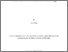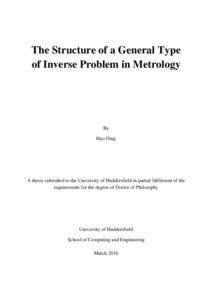Ding, Hao (2016) The Structure of a General Type of Inverse Problem in Metrology. Doctoral thesis, University of Huddersfield.
Abstract

Inverse problems are ubiquitous in science. The theory and techniques of inverse problems play important roles in metrology owing to the close relation between inverse problems and indirect measurements. However, the essential connection between the concepts of inverse problems and measurement has not been deeply discussed before. This thesis is focused on a general type of inverse problem in metrology that arises naturally in indirect measurements, called the inverse problem of measurement (IPM).
Based on the representational theory of measurement, a deterministic model of indirect measurements is developed, which shows that the IPM can be taken as an inference process of an indirect measurement and defined as the inference of the values of the measurand from the observations of some other quantity(s). The desired properties of solving the IPMs are listed and investigated in detail. The importance of estimating empirical relations is emphasised. Based on the desired properties, some structural properties of the IPMs are derived using category theory and order theory. Thereby, it is demonstrated that the structure of the IPMs can be characterised by a notion in order theory, called ‘Galois connection’.
The deterministic model of indirect measurements is generalised to a probabilistic model by considering the effects of measurement uncertainty and intrinsic uncertainty. The propagation of uncertainty from the observed data to the values of measurands is investigated using a method of covariance matrices and a Bayesian method. The methods of estimating empirical relations with probability assigned using the solutions of IPM are discussed in two different approaches: the coverage interval approach and the random variable approach.
For estimating empirical relations and determining the conformity of measurement results in indirect measurements, a strategy of estimating the empirical relations with high resolution is developed which significantly reduced the effect of measurement uncertainty; a method of estimating specification uncertainty is proposed for evaluating the intrinsic uncertainties of measurands; the impact of model resolution on the specifications of the indirectly measured quantities is discussed via a contradiction in the specifications of surface profiles.

Information
Library
DocumentsPreview
Final thesis - DING.pdf - Accepted Version